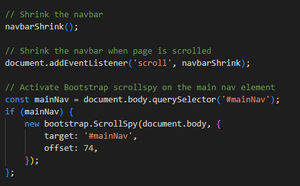Open In App

In a program, comments are like indents one makes, they are used so that it is easier for someone who isn’t familiar with the language to be able to understand the code. It will also make the job easier for you, as a coder, to find errors in the code since you will be easily able to find the location of the bug. Comments are ignored by the compiler while compiling a code, which makes the job more complex in the long run when they have to go through so much code to find one line.

### In Java there are three types of comments:

A beginner-level programmer uses mostly single-line comments for describing the code functionality. It’s the easiest typed comments.

Syntax:

`//Comments here( Text in this line only is considered as comment )`

Illustration:Examples in an actual code

Example:

## Java

 `// Java program to show single line comments`` ` `class` `Scomment``{``    ``public` `static` `void` `main(String args[])``    ``{ ``         ``// Single line comment here``         ``System.out.println(``"Single line comment above"``); ``    ``}``}`

Output:

`Single line comment above`

To describe a full method in a code or a complex snippet single line comments can be tedious to write since we have to give ‘//’ at every line. So to overcome this multi-line comments can be used.

Syntax:

```/*Comment starts
continues
continues
.
.
.
Comment ends*/```

Example:

## Java

 `//Java program to show multi line comments``class` `Scomment``{``    ``public` `static` `void` `main(String args[])``    ``{ ``        ``System.out.println(``"Multi line comments below"``);``        ``/*Comment line 1``          ``Comment line 2 ``          ``Comment line 3*/``    ``}``}`

Output:

`Multi line comments below`

We can also accomplish single line comments by using the above syntax as shown below:

`/*Comment line 1*/`

This type of comment is used generally when writing code for a project/software package, since it helps to generate a documentation page for reference, which can be used for getting information about methods present, its parameters, etc. For example, http://docs.oracle.com/javase/7/docs/api/java/util/Scanner.html is an auto-generated documentation page that is generated by using documentation comments and a javadoc tool for processing the comments.

Syntax:

```/**Comment start
*
*tags are used in order to specify a parameter
*HTML tags can also be used
*such as <h1>
*
*comment ends*/```

## Java

 `/*package whatever //do not write package name here */`` ` `import` `java.io.*;`` ` `class` `GFG {``    ``public` `static` `void` `main (String[] args) {``        ``/** ``        ``comment line 1 ``        ``comment line 2 ``        ``*/``    ``}``}`

Available tags to use:

Implementation:

## Java

 `// Java program to illustrate frequently used ``// Comment tags`` ` `/**``*

Find average of three numbers!

``* The FindAvg program implements an application that``* simply calculates average of three integers and Prints``* the output on the screen.``*``* @author  Pratik Agarwal``* @version 1.0``* @since   2017-02-18``*/``public` `class` `FindAvg ``{``    ``/**``    ``* This method is used to find average of three integers.``    ``* @param numA This is the first parameter to findAvg method``    ``* @param numB  This is the second parameter to findAvg method``    ``* @param numC  This is the second parameter to findAvg method``    ``* @return int This returns average of numA, numB and numC.``    ``*/``    ``public` `int` `findAvg(``int` `numA, ``int` `numB, ``int` `numC) ``    ``{``        ``return` `(numA + numB + numC)/``3``;``    ``}`` ` `    ``/**``    ``* This is the main method which makes use of findAvg method.``    ``* @param args Unused.``    ``* @return Nothing.``    ``*/`` ` `    ``public` `static` `void` `main(String args[]) ``    ``{``        ``FindAvg obj = ``new` `FindAvg();``        ``int` `avg = obj.findAvg(``10``, ``20``, ``30``);`` ` `        ``System.out.println(``"Average of 10, 20 and 30 is :"` `+ avg);``    ``}``}`

Output:

`Average of 10, 20 and 30 is :20`

For the above code documentation can be generated by using the tool ‘javadoc’:

Javadoc can be used by running the following command in the terminal.

`javadoc FindAvg.java`

This article is contributed by Pratik Agarwal. If you like GeeksforGeeks and would like to contribute, you can also write an article using write.geeksforgeeks.org or mail your article to review-team@geeksforgeeks.org. See your article appearing on the GeeksforGeeks main page and help other Geeks.